Practical Geostatistics 2000 Read the reviews Second (and later) Reprint ErrataContact Isobel Clark with new errata or errors

We would like to thank everyone who provided feedback.

Errata from First Edition and First reprint may be found on the original errata pageLatest corrections courtesy of Kathy Flanders at Auburn University in Alabama. Author’s Note [Isobel]: There are stylistic idiosyncrasies that some readers/reviewers disagree with or are irritated by. We make no apologies for these. To conform to another's writing style would be to stultify and/or ossify the tone and content of our book. How's that for pompous?

Preface

Notation

Chapter 1 Introduction

Chapter 2 Why a statistical approach?

Page 11, Section 2.3: missing a ‘2’ in the simplification of the variance calculation. Cross production simplification should read: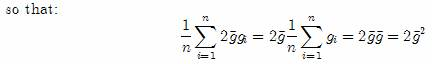Page 16, Section 2.8.1: missing a “÷” sign in the calculation of V, replace second half of page with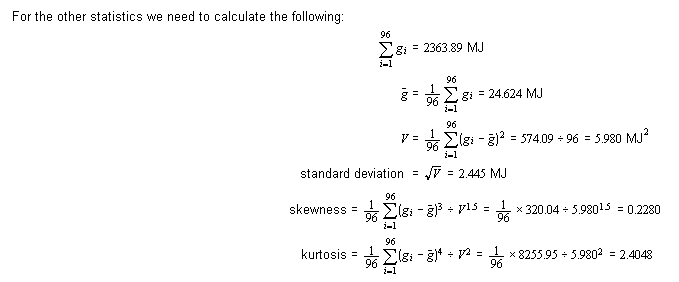Page 17, Section 2.8.1: first sentence after Figure 2.2 should read “From this histogram, we find a modal (highest, most samples) interval between 23.92 and 24.92 MJ.”

Page 21, Section 2.8.3: another division sign problem, this time too many of them!! Skewness and kurtosis calculations should look like this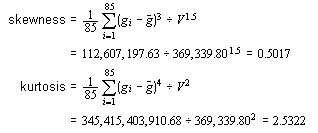Chapter 3 Normal distributions

Page 36, Section 3.2: modified Figure 3.4

Page 41, Section 3.3.2: after definition equation for s@, next line should read “where s@ is now an unbiassed estimator for s@, the true variance of the population.” Throughout this chapter, you should replace “best unbiased estimate for the standard deviation of the population” with “ ‘best’ estimate for…….”.

Page 45, Section 3.4: third line of second paragraph, ‘pay value’ should be ‘pay limit’

Page 55, Section 3.6.2: modified Figure 3.11

Page 59, Section 3.6.3: third bullet down, last sentence should read “That is, 58.5\% of the distribution lies between m ± 0.815s.”

Chapter 4 Lognormal distributions (and others)

Page 71, Section 4.1: that pesky division sign again! Equation for V should read: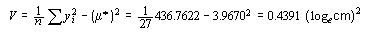Page 79: Section 4.2: typo in the last line of the three parameter calculation. Should read: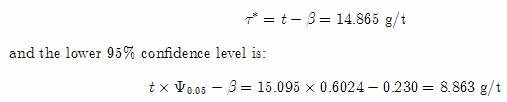Page 80, Section 4.3: last text line should read “the constant should also be added to the values before taking logarithms:”

Page 81, Section 4.3: definition of P’ should read: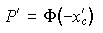Page 84, Section 4.3.2: paragraph starts "From Table 4, F (+1.5056)=0.9339" should read "From Table 1, F (+1.5056)=0.9339". Double apologies for CD readers, as the hyperlink is also to Table 4.

Page 88: slip of the fingers produced an additive constant of 2.8337 instead of the correct one of 2.3887 (sorry!)

Page 92, Section 4.5.2: some major aberrations here guys. Can only assume it was a 3 a.m. section. Many apologies. Table should look like: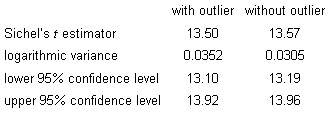and the last paragraph before Section 4.5.3 should just read “It would seem from this table that the outlier has little impact on the Sichel's lognormal estimator.”

Chapter 5 Discrete distributions

Chapter 6 Hypothesis testing

Page 141, Section 6.3.1: there were 22 post graduate students not 23! I must have written this example at 3am because the number of students in both classes is wrong in half a dozen places:

Page 143: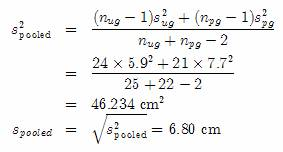and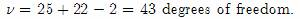Chapter 7 Relationships

Page 149, Section 7.1.1: paragraph just before table, first mention of “graph c” should be “graph b”.

Chapter 8 The Spatial aspect

Page 186, Section 8.1: modified Figure 8.2

Chapter 9 The semi-variogram

Page 208, Chapter 9: modified Figure 9.1

Pages 210-212, Chapter 9: every incidence of ‘121’ should be changed to ‘141’. Apologies for Isobel’s innate stupidity.

Page 211, Chapter 9: calculation of variance at 141/NE. Multiple blunders in one calculation here. All Isobel’s fault, once again. Should read: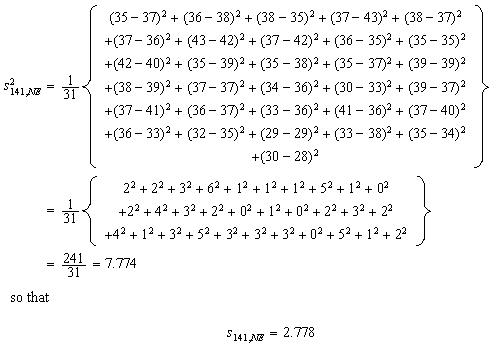Page 212, Chapter 9: “estimated standard deviation of 2.778 %Fe” and confidence interval calculation becomes: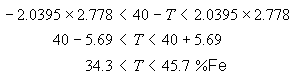Page 218, Section 9.2.3: definition of mixed Spherical model at the bottom of the page, should read: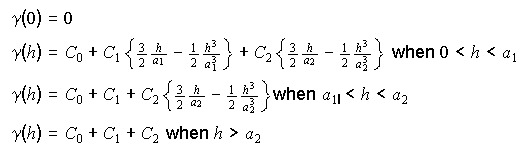Chapter 10 Estimation and Kriging

Yet another apology from Isobel: it has been pointed out to me that many of the ‘hand’ calculations differ from the answers given in the text. This is the same old ‘precision’ problem but largely my fault. For example, on Page 254, the text quotes a distance of 212. In fact, the distance is 150Ï2=212.132 which is what I used in my calculations. If you use 212, you will get noticeable differences. We’ll address this problem in the second edition next year.Spotted by Sven Altfelder.

Page 271, Section 10.2.4: the second equation:

"With a standard error of

ssk = Ï 0.94175 = 0.97 MJ "

" ssk = Ï 0.9177 = 0.96 MJ "

since it is a copy of the last line in section 10.2.1 (Page 269).

Chapter 11 Areas and volumes

Page 296, Chapter 11: Paragraph below Figure 11.1, fourth sentence should read “In the block on the right, we see a block whose value is only 28.8….”

Page 306, Chapter 11: Figure 11.6 horizontal axis should be labelled “cutoff value (lb/ton SnO2)”.

Chapter 12 Other kriging approaches

Bibliography

Tables

Table 1 has been mislabelled. Headings for probability column should read F not f. Apologies for all confusion.

Index